# Excel LAMBDA function: how to write and use

The tutorial explains the Excel LAMBDA function in a simple language and demonstrates a few real-life examples of its use, behavior and possible pitfalls.

Until recently, user-defined functions were the prerogative of programmers. The introduction of LAMBDA has made them available for everyone. What is Excel LAMBDA? In essence, it's a function to create other functions. With this wonderful addition to Excel's toolbox, we no longer need VBA skills to do complex computations, instead we can define our own functions using a familiar formula language. So, let's dive in and get good use out of it!

## Excel LAMBDA function

The LAMBDA function in Excel is designed to create custom functions that can be called by user-friendly names and reused throughout a workbook.

Simply put, you can now take any existing formula, whatever complex it is, wrap it up in LAMBDA, and give it any name you like, say BestFunction. And then, instead of typing your original lengthy formula, you can refer to BestFunction() anywhere in your workbook.

The LAMBDA function works without macros, VBA or JavaScript, so every user and not just programmers can benefit from it.

### What does Lambda mean?

The lambda symbol (λ) representing the 11th letter of the Greek alphabet is often used in physics, mathematics and computer science, where it carries different meanings.

In computer science, the concept of lambdas dates back to lambda calculus (λ-calculus) introduced by American mathematician and logician Alonzo Church in the 1930s. At heart, it is a universal model of computation in which all functions are deemed anonymous and can be formed by abstraction.

Microsoft announced LAMBDA in December 2020 proudly claiming that the new function makes Excel Turing-complete (i.e. computationally universal) and allows users to perform almost any calculations in the native formula language. How is that possible? Due to the fact that a LAMBDA-defined function can call other functions or even itself as many times as needed. This feature is called "recursion", and this is what makes LAMBDA so effective. Earlier, recursion in Excel was only possible through VBA or Office Script.

### Syntax

The Excel LAMBDA function has the following syntax and arguments:

LAMBDA([parameter1, parameter2, …,] calculation)

Where:

Parameter (optional) - an input value that can be supplied in the form of a cell reference, number, or text string. The function accepts up to 253 parameters.

Calculation (required) - the formula to execute or calculation to perform. It must be the last argument and it must return a result.

Here is an example of a custom LAMBDA function in its simplest form: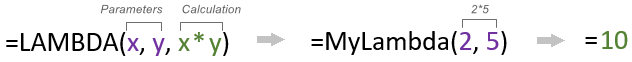### Usage notes

The below guidelines will increase your chances of building a perfectly working custom function at the first attempt and help avoid common errors:

• When naming LAMBDA functions and their parameters, be sure to comply with Excel's standard naming rules.
• A period (.) cannot be used in parameter names.
• For parameters, do not use names that can be confused with cell references. For example, instead of val1 that matches the cell VAL1, use val_1 or value1.
• As with any inbuilt function, follow the best practices for writing formulas: provide the correct number of arguments, match opening and closing parentheses, etc.
• If your LAMBDA function results in an error, these troubleshooting tips will help you detect the problem's root cause.

## What Excel versions have LAMBDA?

The LAMBDA function is only available in Microsoft 365 subscriptions including:

• Excel 365 for Windows
• Excel 365 for Mac
• Excel for the web

## How to write LAMBDA in Excel

To create a Lambda function in your workbook, these are the steps to perform:

### 1. Build a core formula

In most cases, you begin with writing a core formula that returns the desired result. To focus on the process of the LAMBDA creation, we'll keep the formula's logic very simple.

As an example, let's take the classic percentage variance formula:

new_value / old_value - 1

With cell references, it takes this form:

`=C2/B2-1`

To prevent #DIV/0! errors when dividing by a zero value, we wrap it in the IFERROR function like this:

`=IFERROR(C2/B2-1, "-")`

As you can see in the below screenshot, our formula works as intended, so we are ready to move on to the next step: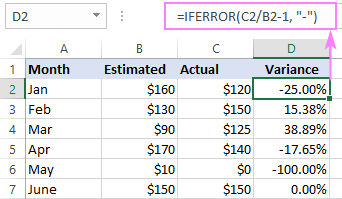### 2. Create and test a LAMBDA formula in a cell

If your formula requires input values, add them as parameters to the LAMBDA function. Our sample formula calculates the percent change between 2 numbers, so we declare 2 parameters:

`=LAMBDA(old, new`

Next, add the formula to the calculation argument. Please notice that instead of cell references we supply the declared parameters:

`=LAMBDA(old, new, IFERROR(new/old-1, "-"))`

If entered in a cell at this point, our formula will return a #CALC! error because it has no values to process. For testing purposes, you should provide the input values in an additional set of parentheses after the formula:

`=LAMBDA(old, new, IFERROR(new/old-1, "-"))(B2, C2)`

This testing syntax allows calling the LAMBDA function from within a cell before naming it:

LAMBDA([param1, param2, …], calculation) (function call)

The screenshot below proves that the results returned by LAMBDA are no different from the original formula: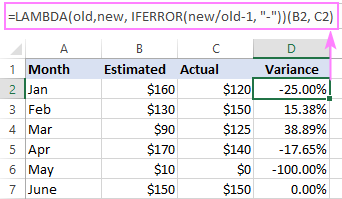### 3. Name the LAMBDA function

After successful testing, you are ready to name your LAMBDA. Here's how:

1. Copy the LAMBDA formula without the function call at the end. In our example, it is:

`=LAMBDA(old, new, IFERROR(new/old-1, "-"))`

2. Open the Name Manager by pressing the Ctrl + F3 shortcut.
3. In the Name Manager dialog, click New.
4. In the New Name dialog box, do the following:
• In the Name box, type the function's name, keeping it short but descriptive.
• Leave the scope set to Workbook (default).
• In the Refers to box, paste the copied formula, ensuring it begins with an equality sign.
• Click OK to save the newly created name.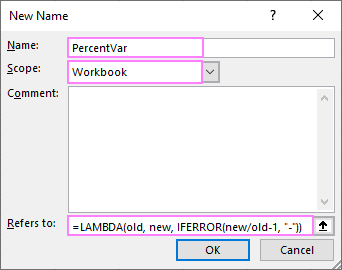Tip. To edit the formula in the Refers to box, press the F2 key to switch from Enter to Edit mode. This will let you navigate through the formula by using the arrow keys without breaking it.

#### LAMBDA naming rules

The syntax rules for LAMBDA names is essentially the same as for other Excel names, please see Excel naming rules.

## How to use LAMBDA in Excel

As soon as your LAMBDA function gets a name, you can refer to it like you would any native function. Our Lambda is named PercentVar and it requires 2 arguments - the old value and the new value:

PercentVar(old, new)

So, we enter the below formula in D2 and copy it down through D7:

`=PercentVar(B2, C2)`

You see, instead of replicating the percent variance formula in its full form, we just feed a couple of input parameters to LAMBDA and get the same results. Perfect!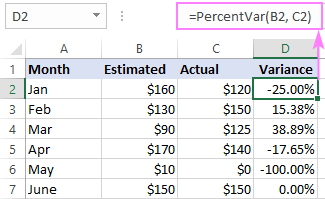## Excel LAMBDA examples

Now that you know the basic concept of LAMBDA in Excel, let's discuss a few more formula examples to really get the hang of it.

### Example 1. Lambda to compact long formulas

LAMBDA is ideal for optimizing long difficult-to-understand formulas.

For example, to get number from any position in string, you can utilize this mind-boggling formula:

`=IF(SUM(LEN(A2)-LEN(SUBSTITUTE(A2, {"0","1","2","3","4","5","6","7","8","9"}, "")))>0, SUMPRODUCT(MID(0&A2, LARGE(INDEX(ISNUMBER(--MID(A2,ROW(INDIRECT("\$1:\$"&LEN(A2))),1))* ROW(INDIRECT("\$1:\$"&LEN(A2))),0), ROW(INDIRECT("\$1:\$"&LEN(A2))))+1,1) * 10^ROW(INDIRECT("\$1:\$"&LEN(A2)))/10),"")`

The person who can construct it from scratch without copy/pasting deserves a Ph.D. in computer science, agree? :)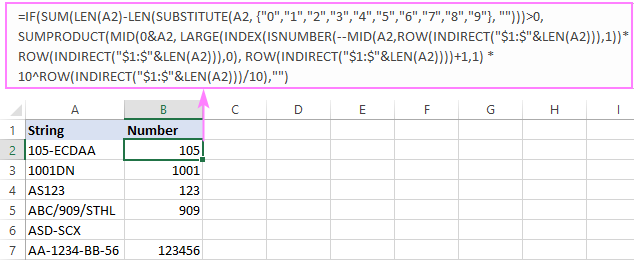Upon a closer look, you may notice that the formula requires just one input value (original string in A2). So, there is nothing that would prevent us from easily converting it into a custom LAMBDA function:

• Firstly, we declare the string parameter, which is the original string from which to extract numbers.
• Secongly, we replace all instances of A2 with string.

That's it!

`=LAMBDA(string, IF(SUM(LEN(string)-LEN(SUBSTITUTE(string, {"0","1","2","3","4","5","6","7","8","9"}, "")))>0, SUMPRODUCT(MID(0&string, LARGE(INDEX(ISNUMBER(--MID(string, ROW(INDIRECT("\$1:\$"&LEN(string))),1))* ROW(INDIRECT("\$1:\$"&LEN(string))),0), ROW(INDIRECT("\$1:\$"&LEN(string))))+1,1) * 10^ROW(INDIRECT("\$1:\$"&LEN(string)))/10),""))`

Once you've made sure that the LAMBDA function works exactly the same as the original formula (for this, use the testing syntax described in the previous section), head over to the Name Manager to define some good name for it, say ExtractNumber.

Voila, you now have a concise and elegant solution to pull numbers from anywhere in a string:

`=ExtractNumber(A2)`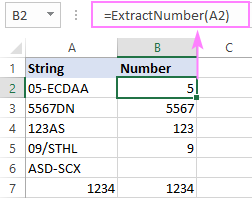### Example 2. LAMBDA with multiple parameters

As mentioned earlier, the LAMBDA function can potentially handle up to 253 parameters. In practice, it would be wise to limit the number of parameters to an absolute minimum because of the following reasons:

• Though custom Lambdas do appear in the formula intellisense drop down list, there is no tooltip showing the arguments - a very convenient feature we've got used to with the inbuilt functions. With LAMBDA, it's your responsibility to remember how many arguments are required and supply them in the appropriate order.
• All parameters are optional. What's wrong with that, you may ask? Nothing unless just one of many arguments needs to be specified for a given task, e.g. =MyLambda(,,,,"needed param",…). Good luck guessing which one that is :)

For this example, we are going to create a custom function to calculate compound annual growth rate based on this generic CAGR formula:

(EV/BV)^(1/N)-1

Which requires 3 input values:

• BV - Beginning value of the investment
• EV - Ending value of the investment
• N - Number of periods

Converted to LAMBDA, the formula looks as follows:

`=LAMBDA(BV, EV, N, (EV/BV)^(1/N)-1)`

After testing, we go to the Name Manager to name our CAGR function. And here, it makes sense to leave some tips for yourself, and especially for other users of your workbook, explaining what each parameter is. The Comments section is a perfect place for this: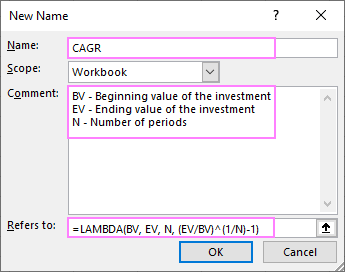Now, we have our own CAGR function for calculating compound annual growth rate in Excel:

CAGR(BV, EV, N)

With the beginning value in E2, ending value in E3, and the number of periods in E4, the formula is as simple as this:

`=CAGR(E2, E3, E4)`

And it works beautifully: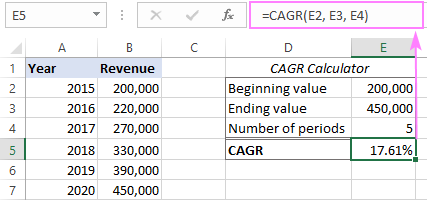On second thoughts, however, it appears that the N parameter is not actually needed in our case. If all the investments are listed in a column like in the image above, then we can have the number of periods calculated automatically with the help of the ROW function:

(EV/BV)^(1/(ROW(EV)-ROW(BV)))-1

In the result, our Lambda becomes a little longer but gets rid of a superfluous argument:

`=LAMBDA(BV, EV, (EV/BV)^(1/(ROW(EV) - ROW(BV))) - 1)`

And now, you only need to provide the beginning and ending values of the investment:

`=CAGR(B2, B7)`

Two is better than three, eh? :)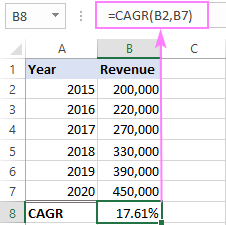### Example 3. LAMBDA with dynamic arrays

Since the LAMBDA function was designed for Excel 365, which is sometimes called Dynamic Array Excel, these two features wonderfully get along.

To see how it works in practice, let's define a custom function to sort a list by the item count. For this, we'll be using the SORTBY function in conjunction with COUNTIF and UNIQUE.

Assuming the items to be sorted are in C2:C85, the formula takes this shape:

`=SORTBY(UNIQUE(C2:C85), COUNTIF(C2:C85, UNIQUE(C2:C85)), -1)`

The logic is quite easy to follow:

• First, we pull all the unique items from the original list: UNIQUE(C2:C85). This array of unique values goes to the 1st argument of SORTBY (array).
• Next, we count how many times each item occurs in the original list: COUNTIF(C2:C85, UNIQUE(C2:C85)). These counts go to the 2nd argument (by_array).
• For the 3rd argument (sort_order), we use -1 to sort descending.

As the result, our SORTBY formula sorts the list of unique items by the item count and arranges the results from highest to lowest.

With the help of this formula, we get a list of tennis Grand Slam winners in the 21st century sorted by the number of wins. To verify the results, you can return the wins count for each champion by using this formula:

`=COUNTIF(C2:C85, E2#)`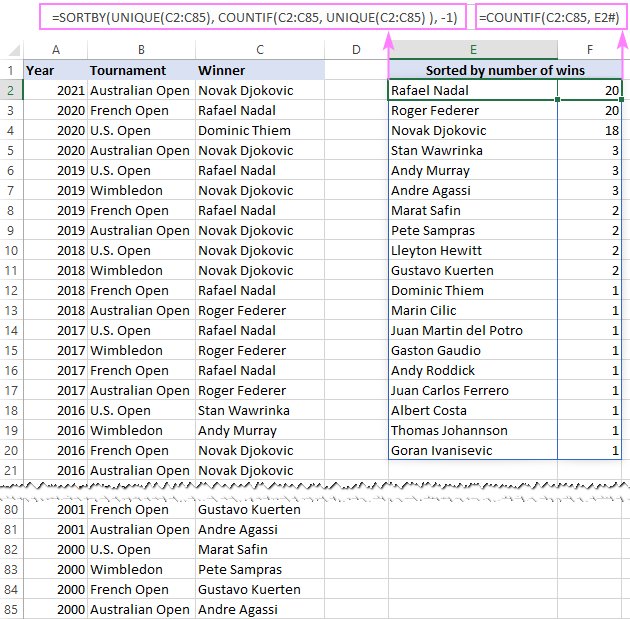Having confirmed that the formula works right, we wrap it up in LAMBDA, replacing the range reference with the parameter name (list):

`=LAMBDA(list, SORTBY(UNIQUE(list), COUNTIF(list, UNIQUE(list)), -1))`

Finally, we name our newly defined function SortByCount, and can now do the same computation with this short and intuitive formula:

`=SortByCount(C2:C85)`

Like any dynamic array formula, it only needs to be entered in a single cell (E2) and returns multiple values into neighboring cells automatically.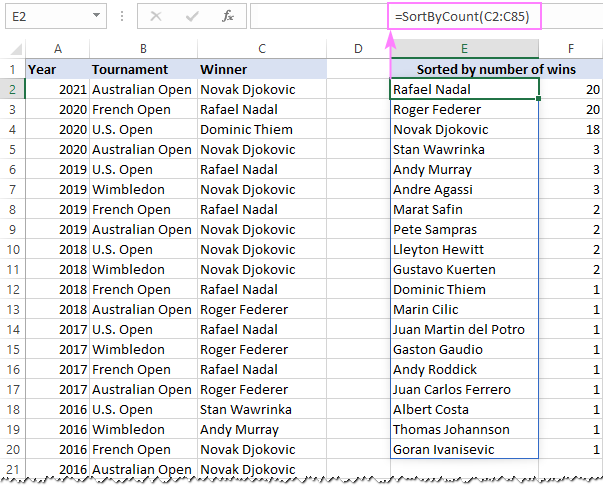To return a limited number of items, say top 3, top 5, or top 10, you can wrap the SORTBY formula in the INDEX function and use an array constant like {1;2;3} to determine the size of an output array, the 3 most referenced items in our case.

`=LAMBDA(list, INDEX(SORTBY(UNIQUE(list), COUNTIF(list, UNIQUE(list)), -1), {1;2;3}))`

Our new Lambda function is named TopThree, and it does exactly what its name suggests:

`=TopThree(C2:C85)`## How to export / import LAMBDA to another workbook

Like anything defined in Excel's Name Manager, LAMBDA is limited to the workbook it is created in.

Luckily, there is quite an easy way to transfer LAMBDA to another workbook. You simply copy a blank sheet from the old workbook to the new one. As the function was saved in the scope of Workbook, it travels with absolutely any worksheet that you copy or move.

Please note that this method exports absolutely all LAMBDA functions that exist in the original workbook.

Hopefully, these examples have inspired you to look for your own uses of LAMBDA in Excel. For now, let me briefly summarize the key takeaways.

## 3 awesome benefits of LAMBDA

If you are still in doubt if the LAMBDA function is something you really need in your workbooks, here are the three compelling reasons to start using it:

1. Instead of cumbersome, hard-to-read formulas, you use compact and elegant functions with descriptive names that you choose.
2. Rather than updating every formula in a workbook, you edit your Lambda function in one place (Name Manager) - a huge improvement that will save you enormous time!
3. Many complex tasks that before could only be solved with VBA can now be accomplished with formulas. This means you don't need to save such workbooks as macro-enabled .xlsm files nor to bother with enabling macros. This mostly becomes possible due to recursive Lambdas, which is the subject of our next tutorial.

## 3 biggest limitations of LAMBDA

As the LAMBDA function is newly released, it's only natural that it has a few rough edges. Here, we'll mention the most essential drawbacks:

1. LAMBDA is not backward compatible. It is only available in Excel 365 and won't work in earlier versions.
2. Lambda functions are workbook-specific and cannot be reused across different workbooks. This might cause confusion in a situation when, in different files, you create Lambdas with the same names but slightly different syntax, and they produce different results for the same input data.

To transfer a LAMBDA-defined function from one workbook to another, you can copy any sheet from the workbook containing the LAMBDA of interest. Then you can delete the copied sheet, but the LAMBDA function will still remain in the Name Manager. Please keep in mind that this method copies all LAMBDAs from the original workbook even if the copied sheet does not contain a single LAMBDA reference.

3. Custom Lambdas do not show tooltips for arguments, so you'll have to memorize the parameters required for each function. As a workaround, you can add a brief description of each parameter in the Name Manager comments, and they will be displayed as you start typing the function's name in a cell (thank you David for this useful tip!):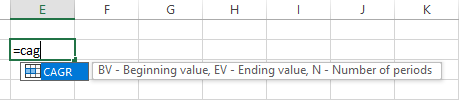## Excel LAMBDA function not working

If you are facing problems while defining a Lambda or your formula throws an error, the following information can help you pin down the cause and fix it.

### #NAME! error

May occur because of the following reasons:

• Your Excel version does not support LAMBDA - currently it is only available to Microsoft 365 users. If you have a Microsoft 365 subscription, make sure the latest Office version is installed on your computer. For more details, please see Excel LAMBDA availability.
• The name of your custom Lambda function is mistyped in a cell.

### #VALUE! error

May be caused by one of these issues:

• When writing a Lambda function, the names used in calculation do not match the declared parameters.
• When entering a formula in a cell, you've specified an incorrect number of arguments - double check the syntax of your Lambda function in the Name Manager.
• Least likely, more than 253 parameters are declared. (I cannot imagine such a function, but in theory this could happen :)

### #NUM! error

Occurs because of a circular call of LAMBDA from within itself, e.g. when a recursive Lambda function does not have a way to exit the loop.

### #CALC! error

May be triggered when creating a new LAMBDA function in a cell without providing input values for testing. To avoid the error, use a special testing syntax described in How to create and test a LAMBDA formula.

### Invalid parameter names

When a seemingly simple LAMBDA function you are creating fails, the problem may be in invalid parameter names that can be confused with cell references. In such cases, Excel highlights the parameters like shown in the screenshot below and throws You've entered too few arguments for this function error: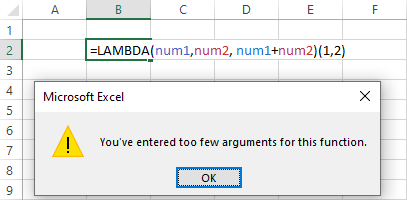The point is that the strings num1 and num2 match the cell addresses NUM1 and NUM2, and therefore Excel does not accept them as parameter names. Once you change the names, say, to num_1 and num_2­, the error disappears, and the LAMBDA function starts working as expected: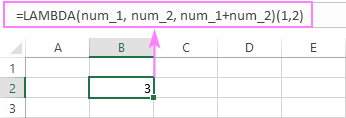The bottom line: if Excel highlights the parameters of a Lambda function you are creating, try different param names that do not look like cell references.

That's how to write and calculate LAMBDA in Excel. In the next article, we'll look at how to create even more powerful recursive functions. Thank you for reading and please stay tuned!

Excel LAMBDA function examples (.xlsx file)

## You may also be interested in:

1. Re: Exporting a Lambda

I've noticed you can import a Lambda from another workbook simply by copy/pasting a cell that contains the function from the source to the destination workbook.

I'm still hoping the Excel team will give us an option comparable to the 'organizer' feature in Word where we could import the Lambdas from any open workbook or even choose a closed workbook to pull from.

2. @Svetlana Cheusheva: I have been learning a lot from your blog. Everyday I am enhancing my Excel knowledge. Bunch of thanks for you..

3. Hi,
Thanks for a very good site!
What would you say is the most resource-effective formula for building an index from percentage changes?
Assume you have percentage changes of a any variable (e.g. daily changes in a stock price) in A2:A1000 for instance in the form of a dynamic array, and you want to build an index starting at 100 in column B. In its simplest form, you would enter 100 in B1, enter B1*(1+A2) in B2 and copy that formula down to (in this case) B1000. But how do you suggest to do this in the most resource effective way, so that B1:B1000, or at least B2:B1000 becomes a dynamic array following the length of A2#, i.e. if A2# is 2345 rows, B1# becomes 2346 rows?
Thanks a million for any tips!
Kindly,
Johan

• The best (I think) I can come up with on my own is:
=SCAN(\$B\$1;B2#;LAMBDA(ix;chg;ix*(1+chg))) in B2# and 100 in B1
I don't know how resource-efficient it is, though...

4. Issue I'm experiencing: Any time I add a Lambda formula to a cell it requires all other users in the shared spreadsheet to reopen the file.

In our workplace we all run off a central spreadsheet that among other things determines ordering dates (I'm the purchaser).
I've created a Lambda formula that will take the start fabrication date, calculate the order time based off lead time for that time of product, and ensure the order date doesn't fall on a weekend or statutory holiday (using the recursive function in Lambda)

So in the cells that get order dates I have the nice and easy to use "=orderDate"
orderDate (calculated date that is neither a weekend or a stat holiday) =IF(SFD="","",recurse(sub,daysOff))
SFD (start fab date) = a date value from another column in the same row
recurse (formula that prevents order date from being a weekend) =LAMBDA(x,y,IF(OR(x=y,WEEKDAY(x)=1,WEEKDAY(x)=7,recurse(x-1,y),x))
daysOff (column that contains all the stat-holidays in the next year) =OFFSET(daysOff!\$A\$1,,,COUNTA(daysOff!\$A:\$A),1)

Any time the formula "=orderDate" is typed/pasted/written with VBA into a cell, it will force every other user to reopen the spreadsheet.
Is this a "feature" that I can expect with ANY Lambda formula in excel? Is this something buggy with the way I've constructed these fomulae? I'm pulling my hair out trying to figure out why this happens, and it doesn't seem to be something that anybody else has ever had an issue with (from any of the google searches I've done)

5. This is my formula =IF(COUNTIF(\$D\$2:D403,D403)=1,1,0).
If transactions are duplicate then 0 else 1
How we fixed the cell in Lambada?

Emp_ID | Counta
1 | 1
1 | 0
2 | 1
3 | 1
3 | 0

6. Hello colleagues

I have three columns with ID, flow and contributing basins, the basin's column are separated by commas (many values), the question is how to search for the basins by ID and replace it with the corresponding flow and add it to another column, thank you very much.

7. Are parameters passed as values or references? I have a lambda() that includes an index() against a large array: 22 columns x 8,000 rows. Since I cannot implicitly specify the array area within the lambda(), I must pass the entire thing. The page seems to run quickly enough, but I am hoping for a better way to do this, especially if the entire array is being passed.

And no, I prefer to not do it as a map() because each row requires specific processing (name, birth date, age, school year...). The sample below is simplified, but the spreadsheet has more processing depending upon the type of data.

sample:

fromMaster = LAMBDA(sourceArray,masterMatch,increment,
LET(
startOfSet,(INT((masterMatch)/22)*22)+7,
personCol,(masterMatch-startOfSet)+1,
INDEX(sourceArray,startOfSet+increment,personCol))
)
;

• sorry: explicitly, not implicitly

• Never mind. I used map()s instead. The defined lambda() became too cumbersome.
The lambda() within a map() allows referencing outside the passed parameters, so that became the better way to do this.
Still, do you know if parameters are passed as values or references to a defined lambda?

8. is there a way to convert a lambda name to the formula it represents?

i.e. =lambda(x,y,x*y)
name = multiply
=multiply(2,2) would then turn into =2*2

• Hi!

Please see the "3. Name the LAMBDA function" section of this tutorial.

9. Thank you.
I think you should add 2 more sections:

1. How to import LAMBDAs
2. How to export LAMBDAs

• Hi Meni,

The easiest way to export LAMBDA to a new workbook is to copy any blank sheet from the original workbook in which it was defined. The export / import section with more details is added, thank you for the idea!

10. Hi Svetlana,
There is a typo in "Open the Name Manager by pressing the Ctrl + 3 shortcut". It should be "Ctrl + F3".
Just my 2 cents. I love your articles.
Rodolfo

• Thank you, Rodolfo! Fixed :)

11. Interesting.

As I believe, most rules are the same as general Excel Names, as can be found in this article of yours:

(also, I think you should mention the "LET function" at the start of this article, or integrate it somewhere else in the main body, and talk about the relations of it with the "LAMBDA function", differences, and the possibility to use them together.)

• Hi Oz,

You are right - naming rules for the LAMBDA function follow the same syntax rules as other Excel names. A mini-section with the reference to the corresponding page is added.

As for the LET function, I don't see where it can be smoothly integrated in the post. Perhaps, it makes sense to add a separate example illustrating this. Will give it a thought...

12. Thanks for the useful info.
Appreciate where to find following on Lambda demand on resource e.g.:
⒈ is Lambda volatile?
⒉if yes, how to compare its volality to Indirect function?

• Hi Sunny,

I was not able to find any information about LAMBDA's volatility. My understanding is that it depends on the native functions used in it. If any function in the core formula is volatile, then LAMBDA is also volatile, otherwise it's not.

13. I stumbled on to this site , when I was searching for an example / syntax for Sumif(), thank you for putting in the effort to explain with such simplicity and relatable example. This got me exploring other tips for Excel and found the best and very clear steps for Lambda. I never knew that Excel 365 had this feature
Thank you

• Thank you for your feedback, Antony! It's nice to hear that our articles are helpful​ :)

14. Counter to 2 of your 3 limitations, if you copy a worksheet that contains a LAMBDA reference to another workbook then the LAMBDA travels with it, so you can indeed copy LAMBDA formulas between workbooks. Although the LAMBDA does not include formal tool tips, it does display the comment that you add in the Name Manager before you type the parenthesis, so that's an opportunity to remind oneself of the parameter names.

• Hello David,

Thank you for these very useful remarks, they indeed neutralize 2 of 3 limitations.

Just one thing to note - copying a worksheet that contains a LAMBDA reference to another workbook transfers *all* LAMBDA functions that exist in the source workbook. Even copying a blank sheet from the workbook containing one or more LAMBDA functions transfers all those functions.

Hope you won't mind if I add this information to the tutorial for other users to know. Again, thank you for your feedback!